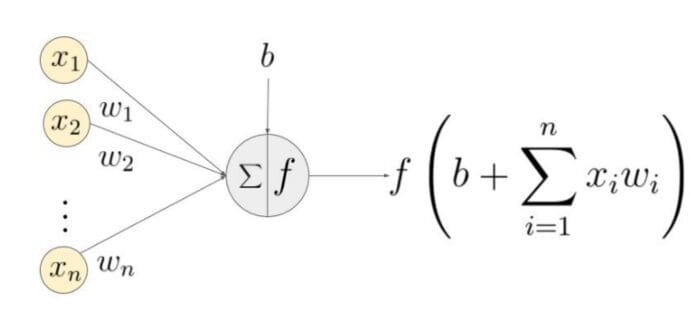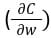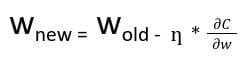This blog post aims to describe the vanishing gradient problem and explain how use of the sigmoid function resulted in it.

The sigmoid function is one of the most popular activations functions used for developing deep neural networks. The use of sigmoid function restricted the training of deep neural networks because it caused the vanishing gradient problem. This caused the neural network to learn at a slower pace or in some cases no learning at all. This blog post aims to describe the vanishing gradient problem and explain how use of the sigmoid function resulted in it.

## Sigmoid function

Sigmoid functions are used frequently in neural networks to activate neurons. It is a logarithmic function with a characteristic S shape. The output value of the function is between 0 and 1. The sigmoid function is used for activating the output layers in binary classification problems. It is calculated as follows:On the graph below you can see a comparison between the sigmoid function itself and its derivative. First derivatives of sigmoid functions are bell curves with values ranging from 0 to 0.25.Our knowledge of how neural networks perform forward and backpropagation is essential to understanding the vanishing gradient problem.## Forward Propagation

The basic structure of a neural network is an input layer, one or more hidden layers, and a single output layer. The weights of the network are randomly initialized during forward propagation. The input features are multiplied by the corresponding weights at each node of the hidden layer, and a bias is added to the net sum at each node. This value is then transformed into the output of the node using an activation function. To generate the output of the neural network, the hidden layer output is multiplied by the weights plus bias values, and the total is transformed using another activation function. This will be the predicted value of the neural network for a given input value.## Back Propagation

As the network generates an output, the loss function(C) indicates how well it predicted the output. The network performs back propagation to minimize the loss. A back propagation method minimizes the loss function by adjusting the weights and biases of the neural network. In this method, the gradient of the loss function is calculated with respect to each weight in the network.

In back propagation, the new weight(wnew) of a node is calculated using the old weight(wold) and product of the learning rate(ƞ) and gradient of the loss function.With the chain rule of partial derivatives, we can represent gradient of the loss function as a product of gradients of all the activation functions of the nodes with respect to their weights.Therefore, the updated weights of nodes in the network depend on the gradients of the activation functions of each node.

For the nodes with sigmoid activation functions, we know that the partial derivative of the sigmoid function reaches a maximum value of 0.25. When there are more layers in the network, the value of the product of derivative decreases until at some point the partial derivative of the loss function approaches a value close to zero, and the partial derivative vanishes. We call this the vanishing gradient problem.

With shallow networks, sigmoid function can be used as the small value of gradient does not become an issue. When it comes to deep networks, the vanishing gradient could have a significant impact on performance. The weights of the network remain unchanged as the derivative vanishes. During back propagation, a neural network learns by updating its weights and biases to reduce the loss function. In a network with vanishing gradient, the weights cannot be updated, so the network cannot learn. The performance of the network will decrease as a result.

## Method to overcome the problem

The vanishing gradient problem is caused by the derivative of the activation function used to create the neural network. The simplest solution to the problem is to replace the activation function of the network. Instead of sigmoid, use an activation function such as ReLU.

Rectified Linear Units (ReLU) are activation functions that generate a positive linear output when they are applied to positive input values. If the input is negative, the function will return zero.The derivative of a ReLU function is defined as 1 for inputs that are greater than zero and 0 for inputs that are negative. The graph shared below indicates the derivative of a ReLU functionIf the ReLU function is used for activation in a neural network in place of a sigmoid function, the value of the partial derivative of the loss function will be having values of 0 or 1 which prevents the gradient from vanishing. The use of ReLU function thus prevents the gradient from vanishing. The problem with the use of ReLU is when the gradient has a value of 0. In such cases, the node is considered as a dead node since the old and new values of the weights remain the same. This situation can be avoided by the use of a leaky ReLU function which prevents the gradient from falling to the zero value.

Another technique to avoid the vanishing gradient problem is weight initialization. This is the process of assigning initial values to the weights in the neural network so that during back propagation, the weights never vanish.

In conclusion, the vanishing gradient problem arises from the nature of the partial derivative of the activation function used to create the neural network. The problem can bevworse in deep neural networks using Sigmoid activation function. It can be significantly reduced by using activation functions like ReLU and leaky ReLU.

Tina Jacob is passionate about data science and believes life is about learning and growing no matter what it brings.